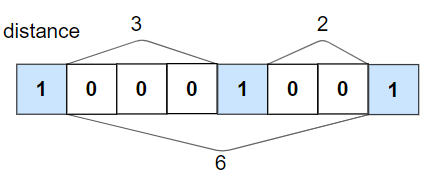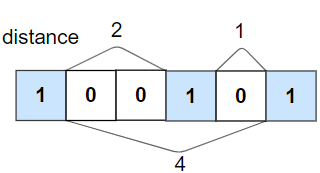# GeetCode Hub

Given an array `nums` of 0s and 1s and an integer `k`, return `True` if all 1's are at least `k` places away from each other, otherwise return `False`.

Example 1:```Input: nums = [1,0,0,0,1,0,0,1], k = 2
Output: true
Explanation: Each of the 1s are at least 2 places away from each other.
```

Example 2:```Input: nums = [1,0,0,1,0,1], k = 2
Output: false
Explanation: The second 1 and third 1 are only one apart from each other.```

Example 3:

```Input: nums = [1,1,1,1,1], k = 0
Output: true
```

Example 4:

```Input: nums = [0,1,0,1], k = 1
Output: true
```

Constraints:

• `1 <= nums.length <= 105`
• `0 <= k <= nums.length`
• `nums[i]` is `0` or `1`

class Solution { public boolean kLengthApart(int[] nums, int k) { } }